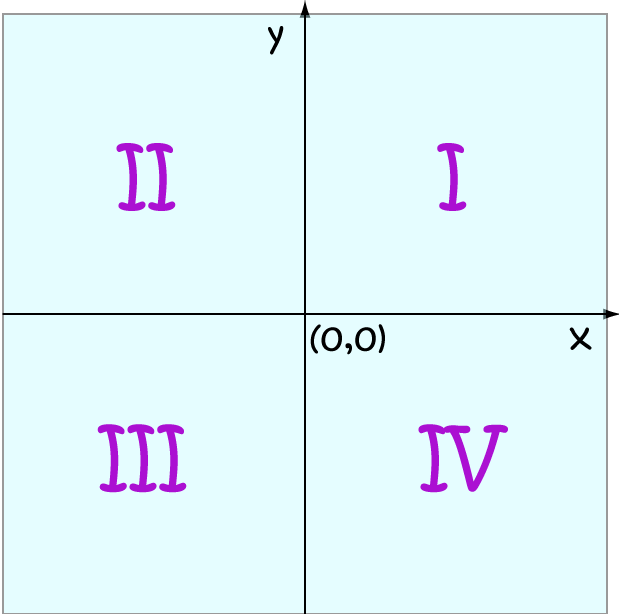# Math Definitions - Letter Q

### ChaptersThe $x$ and $y$-axes divide the plane up into four equal regions called quadrants.

We usually label them using the Roman Numerals, I,II,III and IV:

• In quadrant I, both $x$ and $y$ are positive.
• In quadrant II, $x$ is negative and $y$ is positive.
• In quadrant III, both $x$ and $y$ are negative.
• In quadrant IV, $x$ is positive and $y$ is negative.

### Description

The aim of this dictionary is to provide definitions to common mathematical terms. Students learn a new math skill every week at school, sometimes just before they start a new skill, if they want to look at what a specific term means, this is where this dictionary will become handy and a go-to guide for a student.

### Audience

Year 1 to Year 12 students

### Learning Objectives

Learn common math terms starting with letter Q

Author: Subject Coach
You must be logged in as Student to ask a Question.